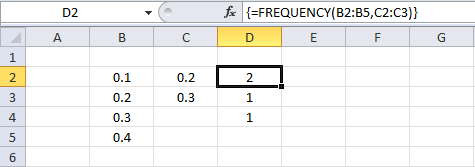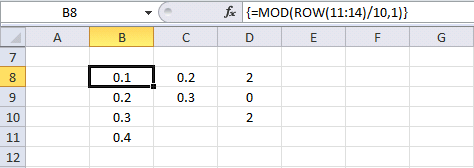Author: Oscar Cronquist Article last updated on July 25, 2017

Can someone explain to me why this happens?

This example is working.FREQUENCY function returns {2; 1; 1}. 2 values (0.1 and 0.2) are equal to or less than 0.2. 1 value (0.3) is larger than 0.2 and equal or smaller than 0.3. 1 value (0.4) is larger than 0.3.

This example is not working as I thought it would.FREQUENCY function returns {2; 0; 2} and I don´t understand why?

The formula in cell B8:B11 returns this array {0.1; 0.2; 0.3; 0.4}, exactly the same values as in B2:B5.

=MOD(ROW(11:14)/10,1)

It seems to be the MOD function but why?

Why am I using the MOD function? To extract the fractional part of a number.

### Get workbook

Frequency bug.xlsx